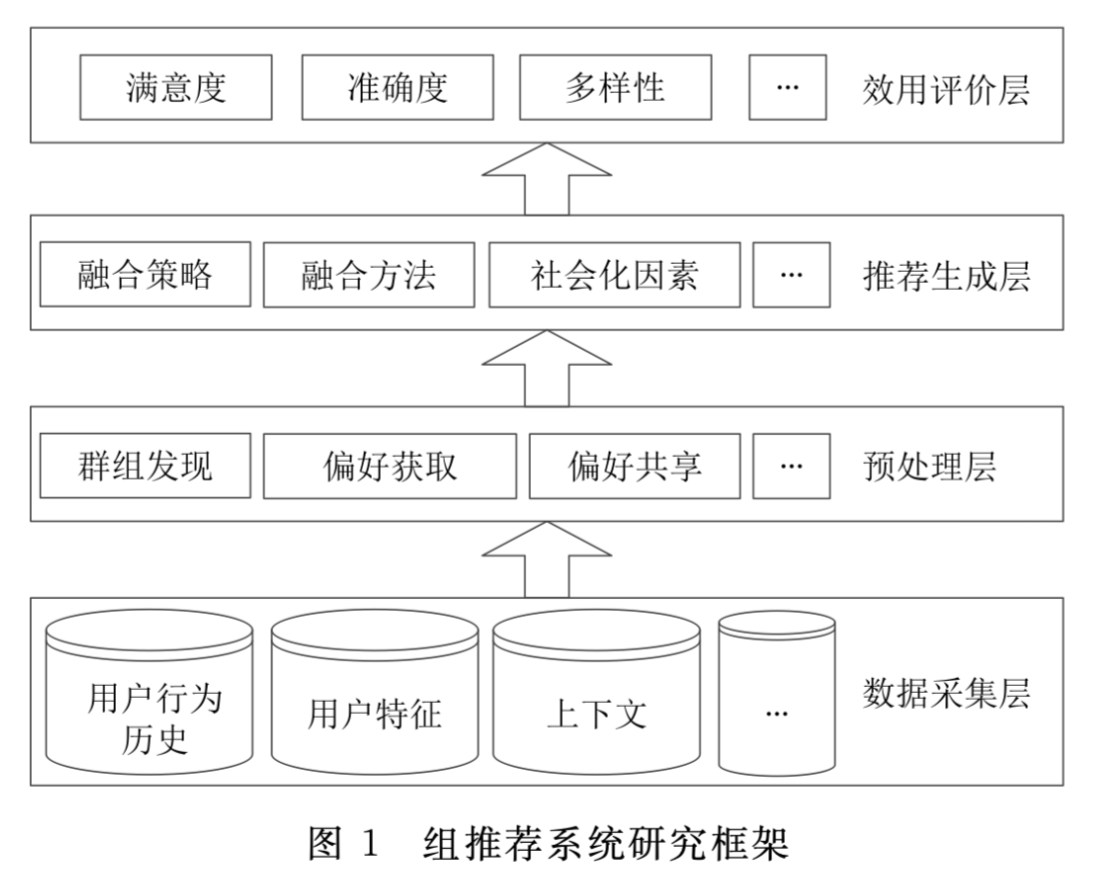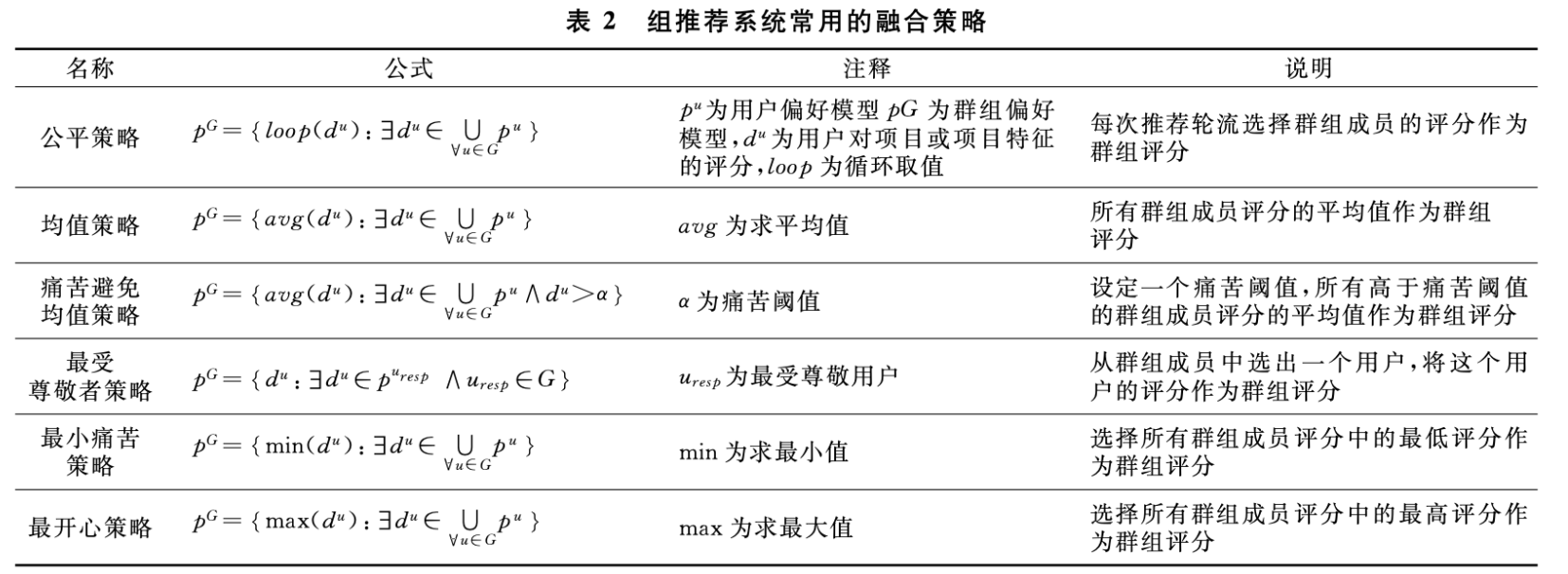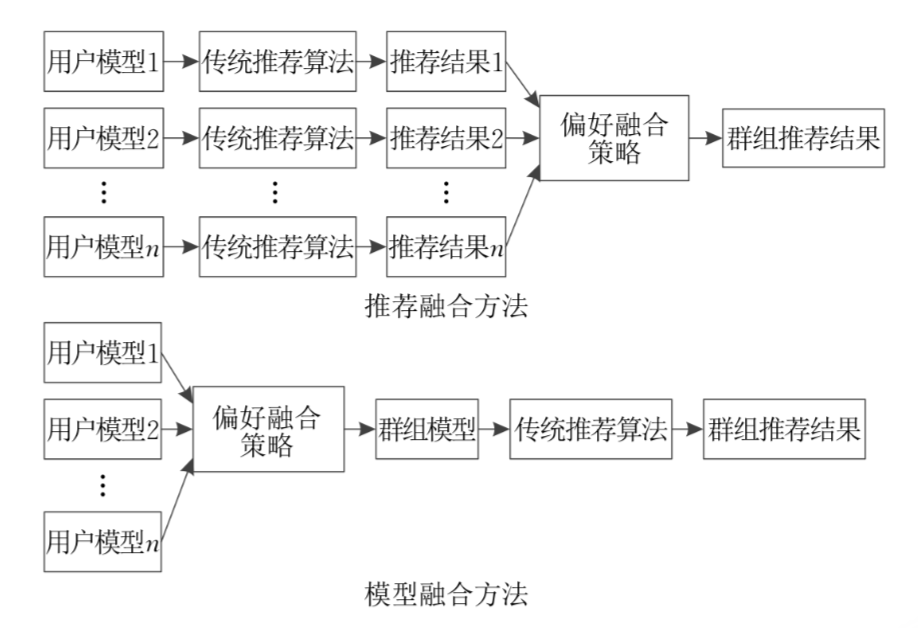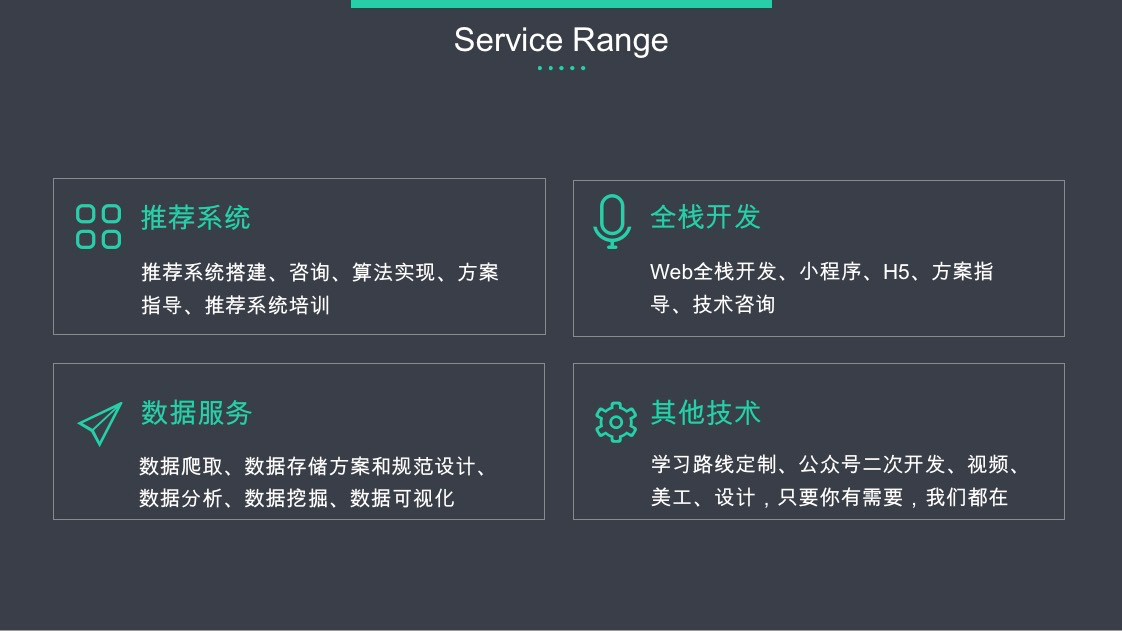## 传统推荐系统

• 从推荐模型的角度：
• 协同过滤推荐
• 基于内容的推荐
• 基于知识的推荐
• 组合推荐
• 基于人口统计学的土建
• 基于效用的推荐
• 基于关联规则的推荐
• 基于网络结构的推荐
• 从推荐系统的数据源和应用环境的角度：
• 上下文感知推荐
• 社会化推荐
• 移动推荐
• 从推荐系统技术的角度：
• 基于规则的推荐
• 基于机器学习的推荐
• 基于深度学习的推荐
• 基于强化学习的推荐
• 基于知识图谱的推荐

## 群组推荐系统的形式化定义

1、群组预测评分

2、群组分歧度

3、共识函数

• $w_1$ 表示群组预测分在共识函数中的权重
• $w_2$ 表示群组分歧度在共识函数中的权重

4、Top-k 组推荐

## 群组推荐与传统推荐的异同

- 传统推荐系统 群组推荐系统

• 常见的协同、基于内容、组合推荐等也会用在群组推荐中
• 群组推荐也可以作为一路召回为单个用户推荐
• 群组推荐可以帮助解决“用户冷启动”问题

## 群组推荐系统研究框架

• 收集群组成员数据
• 获取群组成员偏好信息
• 生成群组推荐
• 推荐结果的评价和反馈• 群组成员行为历史
• 群组成员浏览记录
• 群组用户-项目评分
• 群组成员人口统计学特征
• 项目特征
• 上下文信息

• 用户满意度
• 准确度
• 覆盖率
• 多样性
• 精细度

## 群组推荐的关键技术

### 用户偏好获取

• 显式反馈：能明确表示用户对内容喜好等级的数据，比如评分，打星等
• 隐式反馈：用户与物品之间产生的交互行为，没有明确的表示喜好等级

### 群组推荐的群组发现

• 层次化聚类
• 划分式聚类
• KMeans聚类
• 二分KMeans聚类
• 基于滑窗的聚类
• Mean-Shift 聚类
• 基于密度的聚类
• DBSCAN
• 基于网格的聚类
• 新发展的一些方法
• 基于约束（eg：EM聚类）
• 基于模糊
• 基于粒度
• 量子聚类
• 核聚类
• 谱聚类

### 群组推荐的偏好融合算法

#### 偏好融合策略• 静态模型：根据群组成员的年龄、性别等人口统计学信息对用户进行分类 然后对不同类别赋予不同的权重，比如：一个针对00后的社区对青年和老年人分配较小的权重
• 交互模型：根据用户与内容之间的交互行为来分配权重。比如：用户的行为越多，说明用户越活跃，则权重越大

• The role-based model

$role(u_y)=role(u_x)$表示和用户$u_x$角色相同的用户

• The family-log model

Aggregation Strategies（https://dl.acm.org/doi/10.1145/1864708.1864732）

• $r_1$表示最小痛苦策略的最小阈值
• $r_2$表示最开心策略的最大阈值
• $rat(u_x, item_i) \notin (r_1, r_2)$表示 $u_x$对$item_i$的评分不在$(r_1, r_2)$时权重为1，其他为$w(u_x, f_a)$

#### 偏好融合方法

• 推荐结果融合
• 评分融合
• 群组偏好建模

• 模型融合
• 推荐融合• $rat(g_a, item_i)$表示群组$g_a$对项目$item_i$的评分
• $rat(u_x, item_i)$表示用户$u_x$对项目$item_i$的评分
• $w(u_x, g_a)$表示用户$u_x$在群组$g_a$中的权重
• $pred(g_a, item_n)$ 表示群组$g_a$对未评分项目$item_n$的预测评分
• $sim(g_a, g_b)$表示群组$g_a$和$g_b$的偏好相似度

Aggregation Strategies（https://dl.acm.org/doi/10.1145/1864708.1864732）

#### 群组推荐的评价

• 准确度：RMSE、MAP、F1、E(k)、Recall、Precision、AUC、NDCG
• 用户满意度
• 多样性
• 覆盖率
• 惊喜度

## 群组推荐研究的难点和发展方向

• 基于内存
• 基于模型

### 群组推荐中存在传统推荐问题

OK，普及完毕！

【技术服务】，详情点击查看： https://mp.weixin.qq.com/s/PtX9ukKRBmazAWARprGIAg